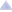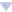### awoo's blog

By awoo, history, 4 weeks ago,1861A - Prime Deletion

Idea: BledDest

Tutorial
Solution (BledDest)

1861B - Two Binary Strings

Idea: Roms

Tutorial
Solution (Roms)

1861C - Queries for the Array

Idea: BledDest

Tutorial
Solution 1 (Roms)
Solution 2 (BledDest)

1861D - Sorting By Multiplication

Idea: Roms

Tutorial
Solution (Roms)

1861E - Non-Intersecting Subpermutations

Idea: BledDest

Tutorial
Solution (BledDest)

1861F - Four Suits

Idea: BledDest

Tutorial
Solution (BledDest)Comments (48)
 » 4 weeks ago, # | ← Rev. 3 →   These solutions explain very clearly.I'm sad I didn't AC problem D, but it is interesting!
 » thanks for the tutorial
 » Good problems, thanks for the round!
 » 4 weeks ago, # | ← Rev. 2 →   "BledDest is a graph theory lunatic" had me cracked up.
•  » » The point is that the sentence's coming from himself.
•  » » Graph theory solution for a greedy problem in author's solution, are you retarded????????????? $\color{white}{just kidding lol}$
•  » » » 3 weeks ago, # ^ | ← Rev. 2 →   First one problem can have multiple solutions. Second why C is greedy problem
 » Test 3,682nd test case->great work in testing!
 » D was looking hard but it is easy
 » Another easy way to implement E is to just combine the last two states into one. So $dp_{i,j}$ counts how many of arrays of length $i$ exist such that the number of elements on the suffix we use is $x \equiv j \pmod k$ and the current cost of the array is $c = j\ /\ k$. Then the transitions are the same, but you just reset the partial sum whenever $j \equiv 0 \pmod k$ since you can never transition to a lower cost.Submission: 221342327
•  » » Thanks this is really helpful
 » 4 weeks ago, # | ← Rev. 2 →   In problem C, if you have : ++++1-0 when we verify the number elements in the array we have 3 elements but the array has to be sorted.Edit : I misunderstood the second condition
 » My man awoo is there just for the vibes; posts both the announcement and tutorial blogs but doesn't author any problem or solution.
 » during contest i had some trouble implementing C (i had same idea as described in approach 1) and using segment trees seemed easier to me. Idea is identical as described in approach 1 but it was easier for me to implement this way, so i thought of sharing it: code.
 » #include using namespace std; #define all(v) (v).begin(), (v).end() void solve(); signed main() { ios_base::sync_with_stdio(false); cin.tie(NULL); cout.setf(ios::fixed); cout.precision(10); srand(time(NULL)); long long t; cin >> t; while (t--) { solve(); } } void solve() { string s; cin >> s; long long n = s.length(); if (n == 1) { if (s == '0' || s == '-') cout << "NO" << endl; else cout << "YES" << endl; return; } long long flag0 = 0; long long flag1 = 1; long long count = 0; for (long long i = 0; i < n; i++) { if (s[i] == '+') { if (flag0 > 0) flag0++; flag1 = 0; count++; } else if (s[i] == '-') { count--; flag0 = max(0LL, flag0 - 1); } if (s[i] == '0') { if (count <= 1 || flag1) { cout << "NO" << endl; return; } if (flag0 == 0) flag0 = 1; } if (s[i] == '1') { if (flag0) { cout << "NO" << endl; return; } flag1 = 1; } } cout << "YES" << endl; } Can anyone tell me what is wrong with this solution to problem C. Thank you in advance
 » Hi all, it would really help if you can post some insights/hints for the problems of this contest on https://starlightps.org ! Here's a link to the problems: https://starlightps.org/problems/collection/cf-edu-154/. This will help us get more data so users can have a platform to: share/discover hints/insights on various problems find similar problems given insights they struggled with. Check it out if you're interested. Thanks!
 » 4 weeks ago, # | ← Rev. 2 →   void solve() { str s; cin >> s; stack st; int nz = 0; for (int i = 0; i < s.length(); i++) { if (s[i] == '+') { st.push(s[i]); } else if (s[i] == '-') { if (st.empty()) { cout << "NO" << endl; return; } else { if (st.top() == '1' || st.top() == '0') { if (st.top() == '0') nz--; st.pop(); st.pop(); } else st.pop(); } } else { if (st.size() < 2) { if (s[i] == '0') { cout << "NO" << endl; return; } } else { if ((st.top() == '1' && s[i] == '0') || (st.top() == '0' && s[i] == '1')) { cout << "NO" << endl; return; } if (s[i] == '1' && nz != 0) { cout << i << " " << "hello" << endl; cout << "NO" << endl; return; } if (s[i] == '0') { nz++; } if (st.top() == '+') st.push(s[i]); } } } cout << "YES" << endl; } what is the error in this code, getting WA in test 3, for problem C. I have used a stack-based approach.
 » Can someone find out what is wrong with my code problem c 221486376
•  » » Take a look at Ticket 17062 from CF Stress for a counter example.
 » Kevin114514 , How can you make A this much complicated ? His submission (221392540)
 » 3 weeks ago, # | ← Rev. 2 →   awoo,BledDest I think in tutorial of problem E, in the paragraph before the last, you should have written: "difference is: $dp_{i,j,c}$", instead of $dp_{i,j+1,c}$because we have according to the second type of transitions$dp_{i+1,j} <== d_{i,j} + d_{i,j+1} + ... + dp_{i,k-1}$$dp_{i+1,j+1} <== d_{i,j+1} + d_{i,j+2} + ... + dp_{i,k-1}$difference: $dp_{i+1,j}-dp_{i+1,j+1} = dp_{i,j}$ and not $dp_{i,j+1}$I hope I'm not mistaking, but correct me if I'm wrong...thx
•  » » Thank you, this is an error. Will be fixed in a couple of minutes
 » woww clear editorial , tnx awoo , BledDest
 » at problem B wouldnt the example a = 00010000 b = 00011111 give a no?
•  » » ignore the question I didnt read that it ends with 1 and starts with 0
 » thank you for the editorial It was really helpful, I have a question How on earth did you come up with that idea of the last x elements on problem E, You assumed some number and built the dp table on the assumed number, it is my first time to see such a thing I Don't think this sort of things come from practice, and the amazing thing is that most of accepted solutions are the same idea, Is that I'm too dump or you are too smart? Thank You :D
 » Why you most of the times post the editorial late, it is expected that you should post the editorial as soon as the hacking phase in over.
 » 3 weeks ago, # | ← Rev. 2 →   The title for D in the tutuorial text is Russian, not English. awoo fix it pls, thx
 » Roms very clean and readable code. Thanks.
 » Hey Author, I am in trouble to get the Problem B according to me for the test case 1 1000101 1000100 I am not able to find the way to make this same : ( but according to the Author code it is printed YES Can anyone know how to make this equal ??
•  » » According to the question .. both the strings should start with 0 and end with 1's. So your test case is wrong.
 » What's wrong with this solution for C?
•  » » 3 weeks ago, # ^ | ← Rev. 2 →   In your code dealing with '-' : if (!unsorted.empty() && elements < unsorted.back()) unsorted.pop_back(); IF shoule be replaced by WHLIE , for the cases like this : ++++000-1 , you need to pop back more than once.Then your code will get accepted. :)
•  » » » Thanks!
 » Any one could help me I don't know what is the wrong in this solution
•  » » 3 weeks ago, # ^ | ← Rev. 2 →   1++++1-0
•  » » » thanks
 » Can someone please explain, what is the partial sum technique mentioned in E and why is it true?
 » 3 weeks ago, # | ← Rev. 6 →   For problem D can someone explain how can this be sorted in 4 operations? n = 9, a = [10 5 10 9 6 9 8 9 5]
•  » » 3 weeks ago, # ^ | ← Rev. 2 →   l=1,r=2,x=3 30 15 10 9 6 9 8 9 5 l=1,r=4,x=-1 -30 -15 -10 -9 6 9 8 9 5 l=7,r=8,x=2 -30 -15 -10 -9 6 9 16 18 5 l=9,r=9,x=4 -30 -15 -10 -9 6 9 16 18 20 
•  » » » Thanks
 » Problem C Approach 1(Roms) Can anyone explain why we need to check it after each query? After each query, we can just check that the longest sorted prefix is shorter than the shortest non-sorted prefix
•  » » If the current query is '1', then make longest sorted prefix equal current length, which is consistent with the current query. And the shortest non-sorted prefix is consistent with the previous queries. If longest sorted prefix is shorter than the shortest non-sorted prefix, it means the current query is valid based on the previous queries.If the current query is '0', then make shortest non-sorted prefix=min(shortest non-sorted prefix, cur_length), which is consistent with the current query. Then check the inequality.
•  » » » thanks
 » Seems like this editorial doesn't explain why there is no better solution in problem D, which makes prefix with length x negative by several multiplications on negative number
•  » » 11 days ago, # ^ | ← Rev. 3 →   Can anyone prove the solution to Problem D. Why does this approach give an optimal solution? Thanks.
 » 23 hours ago, # |+1.ComplaintFrame { display: inline-block; position: absolute; top: 0; right: -1.4em; } .ComplaintFrame a { text-decoration: none; color: #ff8c00; opacity: 0.5; } .ComplaintFrame a:hover { opacity: 1; } ._ComplaintFrame_popup p, ._ComplaintFrame_popup button { margin-top: 1rem; } ._ComplaintFrame_popup input[type=submit] { padding: 0.25rem 2rem; } ._ComplaintFrame_popup ul { margin-top: 1em !important; margin-bottom: 1em !important; } Why Was My Submission Skipped Despite Submitting First https://codeforces.com/blog/entry/120756
 » Can anyone help me in which test case is this solution going wrong it shows WA on 303 (test case 3)